# Triangle Congruence: SSS

## Construct a triangle with the following sides: 3, 5, and 6.

1. Select the SEGMENT WITH GIVEN LENGTH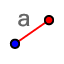tool. Click anywhere in the applet, and enter a length of 3 when asked. This defines the first two vertices of your triangle: points A and B. 2. Select the CIRCLE: CENTER & RADIUS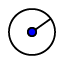tool, and click on point A. Enter a radius of 5 when asked. This shows a set of possible locations for the third vertex of your triangle, which will be point C. 3. With the CIRCLE: CENTER & RADIUStool still selected, click on point B, and enter a radius of 6 when asked. This shows another set of possible locations for the third vertex of your triangle. 4. Select the INTERSECT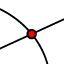tool, and click on a point that is part of both circles. This will be the third vertex of your triangle because it is 5 units from point A and 6 units from point B. 5. Select the POLYGONtool. Then click on A, B, C, and A again. The triangle will turn blue. 6. Select the ANGLEtool, and then click on the three points that define each angle, to make its measure appear. You need to move clockwise across the angle, or the measurement will be more than 180°. If this happens, press undo, and click on the points in reverse order.

What are the angles of any triangle with sides of the following lengths: 3, 5, and 6?

## Use the applet below to answer the questions that follow. If you want, you may use the following instructions to save time.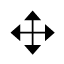1. Select the SLIDER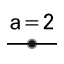tool, and click in a corner of your graph. Change the minimum to 0, and the maximum to 10. Repeat 3 times to create sliders a, b, and c. 2. Select the SEGMENT WITH GIVEN LENGTHtool, and enter c for the length. The enables slider c to control the length of this segment, which is opposite point C. 3. Select the CIRCLE: CENTER & RADIUStool, click on point A, and enter b for the radius. This enables slider b to control the length of the segment that will be opposite vertex B. 4. With the CIRCLE: CENTER & RADIUStool still selected, click on point B, and enter a for the radius. 5. Use the INTERSECTtool, the POLYGONtool, and the ANGLEtool to complete a triangle. Then use the MOVEtool to adjust the sliders to create the triangles below. Click on the MOVE GRAPHICS VIEWtool to reveal more tools that may be useful.

What are the angles of a triangle with sides 2, 6, and 7?

What are the angles of a triangle with sides 2, 3, and 4?

What are the angles of a triangle with sides 3, 5, and 7?

What are the angles of a triangle with sides 3, 5, and 7?